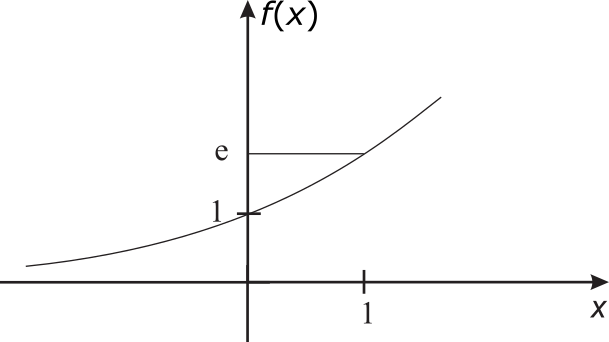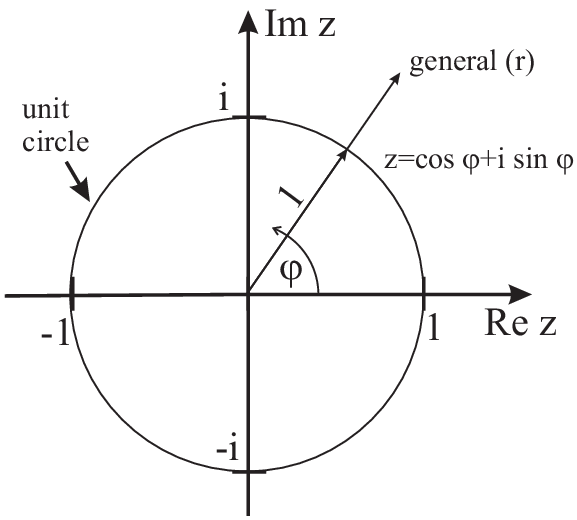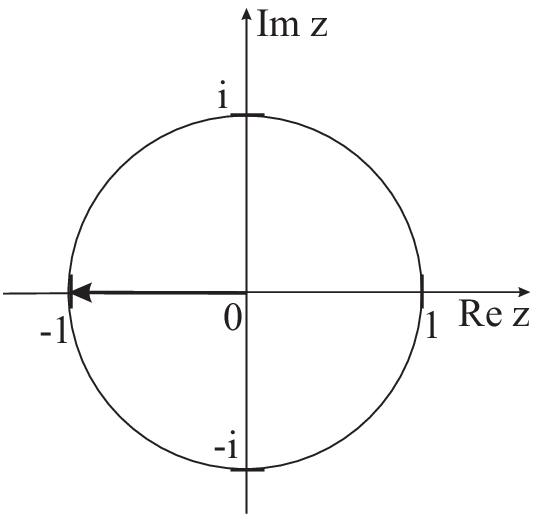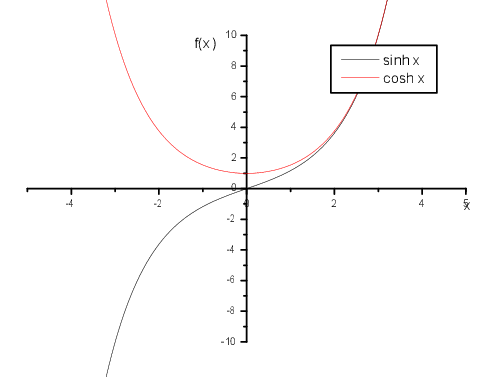### 2.3 Complex e-function

Function:$f\left(x\right)={e}^{x},\phantom{\rule{0.28em}{0ex}}x\in ℝ$ , $\phantom{\rule{0.28em}{0ex}}\phantom{\rule{0.28em}{0ex}}\phantom{\rule{0.28em}{0ex}}\phantom{\rule{0.28em}{0ex}}e=2.7181\dots$We define the exponential function as (the only non trivial function) which is it’s own derivative:
Derivative of the exponential function:

 $\frac{d{e}^{x}}{dx}={e}^{x}$

Just using the definition of the factorial function we find the Taylor series expansion of the exponential function

First we will prove some very important properties of the exponential function.
Fundamental addition formula of the exponential function:
Applying the definition of the e-function as a series we find $\begin{array}{rcll}{e}^{x}{e}^{y}& =& \sum _{n=0}^{\infty }\frac{{x}^{n}}{n!}\sum _{m=0}^{\infty }\frac{{y}^{m}}{m!}& \text{}\\ & =& \sum _{n=0}^{\infty }\sum _{k=0}^{n}\frac{{x}^{k}{y}^{n-k}}{k!\left(n-k\right)!}& \text{}\\ & =& \sum _{n=0}^{\infty }\frac{1}{n!}\underset{k=0}{\overset{n}{\sum }}\left(\genfrac{}{}{0.0pt}{}{n}{k}\right){x}^{k}{y}^{n-k}& \text{}\\ & =& \sum _{n=0}^{\infty }\frac{{\left(x+y\right)}^{n}}{n!}& \text{}\\ & =& {e}^{x+y}& \text{}\end{array}$

Euler’s formula:
taking into account ${i}^{4k}=1,\phantom{\rule{0.28em}{0ex}}\phantom{\rule{0.28em}{0ex}}\phantom{\rule{0.28em}{0ex}}{i}^{4k+1}=i,\phantom{\rule{0.28em}{0ex}}\phantom{\rule{0.28em}{0ex}}\phantom{\rule{0.28em}{0ex}}{i}^{4k+2}=-1,\phantom{\rule{0.28em}{0ex}}\phantom{\rule{0.28em}{0ex}}\phantom{\rule{0.28em}{0ex}}{i}^{4k+3}=-i$ , and using the definitions by the Taylor series we find $\begin{array}{rcll}{e}^{i\phi }& =& \sum _{n=0}^{\infty }\frac{{\left(i\phi \right)}^{n}}{n!}=1+i\phi +\frac{{\left(i\phi \right)}^{2}}{2!}+\frac{{\left(i\phi \right)}^{3}}{3!}+\frac{{\left(i\phi \right)}^{4}}{4!}+\frac{{\left(i\phi \right)}^{5}}{5!}+\dots & \text{}\\ & =& 1-\frac{{\phi }^{2}}{2!}+\frac{{\phi }^{4}}{4!}+\dots +i\left(\phi -\frac{{\phi }^{3}}{3!}+\frac{{\phi }^{5}}{5!}-\dots \right)& \text{}\\ & :=& \mathrm{cos}\phi +i\mathrm{sin}\phi & \text{}\end{array}$

This is a very important relation. It can be understood as the definition of the sin and cos function and allows to replace $\mathrm{cos}\phi$ and $\mathrm{sin}\phi$ by the (complex) e-function (and vice versa) Simplification!!! (e.g. Waves $\stackrel{^}{=}\phantom{\rule{0.28em}{0ex}}$complex $e$ -function). In addition the symmetries of the sin and cos functions get already obvious.

$\mathrm{sin}x$ and $\mathrm{cos}x$ vs. $\mathrm{exp}$:
For real numbers $\mathrm{cos}x$ and $\mathrm{sin}x$ are just the symmetric resp. antisymmetric representation of the $\mathrm{exp}x$ function with the following properties

 ${\mathrm{cos}}^{2}x+{\mathrm{sin}}^{2}x=\left(\mathrm{cos}x+i\mathrm{sin}x\right)\left(\mathrm{cos}x-i\mathrm{sin}x\right)={e}^{ix}{e}^{-ix}=1$

Addition theorems for sin and cos functions:
Combining the exp-addition formula with Euler’s formula we find $\begin{array}{rcll}\left(\mathrm{cos}y\mathrm{cos}z-\mathrm{sin}y\mathrm{sin}z\right)+i\left(\mathrm{cos}y\mathrm{sin}z+\mathrm{sin}y\mathrm{cos}z\right)& =& & \text{}\\ \left(\mathrm{cos}y+i\mathrm{sin}y\right)\left(\mathrm{cos}z+i\mathrm{sin}z\right)& =& {e}^{iy}{e}^{iz}& \text{}\\ & =& {e}^{i\left(y+z\right)}& \text{}\\ & =& \mathrm{cos}\left(y+z\right)+i\mathrm{sin}\left(y+z\right)& \text{}\\ & & & \text{}\end{array}$

From real and imaginary part we finally get (representing the even and odd part of the complex exponential function) $\begin{array}{rcll}\mathrm{cos}y\mathrm{cos}z-\mathrm{sin}y\mathrm{sin}z& =& \mathrm{cos}\left(y+z\right)& \text{}\\ \mathrm{cos}y\mathrm{sin}z+\mathrm{sin}y\mathrm{cos}z& =& \mathrm{sin}\left(y+z\right)& \text{}\\ & & & \text{}\end{array}$

Combining both equations we easily get

 $\mathrm{tan}\left(y+z\right)=\frac{\mathrm{tan}\left(y\right)+\mathrm{tan}\left(z\right)}{1-\mathrm{tan}\left(y\right)\phantom{\rule{0.17em}{0ex}}\mathrm{tan}\left(z\right)}$

Back to complex numbers:

In general:
 $\begin{array}{ccc}z\hfill & \hfill =\hfill & r\left(\mathrm{cos}\phi +i\mathrm{sin}\phi \right)\hfill \\ \text{Re}\left\{z\right\}\hfill & \hfill =\hfill & r\mathrm{cos}\phi \hfill \\ \text{Im}\left\{z\right\}\hfill & \hfill =\hfill & r\mathrm{sin}\phi \hfill \\ r\hfill & \hfill =\hfill & |z|\hfill \end{array}$Multiplication of complex numbers: $\begin{array}{rcll}{z}_{1}\cdot {z}_{2}={r}_{1}{e}^{i{\phi }_{1}}\cdot {r}_{2}{e}^{i{\phi }_{2}}& =& \left({r}_{1}{r}_{2}\right){e}^{i\left({\phi }_{1}+{\phi }_{2}\right)}& \text{}\\ & =& \left({r}_{1}{r}_{2}\right)\left(\mathrm{cos}\left({\phi }_{1}+{\phi }_{2}\right)+i\mathrm{sin}\left({\phi }_{1}+{\phi }_{2}\right)\right)& \text{}\\ z\cdot \stackrel{¯}{z}=r{e}^{i\phi }\cdot r{e}^{-i\phi }& =& {r}^{2}& \text{}\end{array}$

Definition 6

Example:
Has the equation ${e}^{z}=-1$ any solution?Similar to the definition of the cos and sin function we have

Definition 7 hyperbolic functions

 $\begin{array}{rcll}\mathrm{cosh}x& =& \frac{1}{2}\left({e}^{x}+{e}^{-x}\right)\phantom{\rule{2em}{0ex}}\in ℝ& \text{}\\ \mathrm{sinh}x& =& \frac{1}{2}\left({e}^{x}-{e}^{-x}\right)& \text{}\\ \mathrm{tanh}x& =& \frac{\mathrm{sinh}x}{\mathrm{cosh}x}=\frac{{e}^{x}-{e}^{-x}}{{e}^{x}+{e}^{-x}}& \text{}\end{array}$like tan x Definitions also valid for complex arguments $\begin{array}{rcll}\mathrm{sinh}z& =& \frac{1}{2}\left({e}^{z}-{e}^{-z}\right),& \text{}\\ \mathrm{cosh}z& =& \frac{1}{2}\left({e}^{z}+{e}^{-z}\right),& \text{}\\ & & & \text{}\\ \mathrm{sinh}\left(ix\right)& =& \frac{1}{2}\left({e}^{ix}-{e}^{-ix}\right)=i\mathrm{sin}x,& \text{}\\ \mathrm{cosh}\left(ix\right)& =& \frac{1}{2}\left({e}^{ix}+{e}^{-ix}\right)=\mathrm{cos}x& \text{}\end{array}$

Theorem for $\mathrm{cosh}$ and $\mathrm{sinh}$:

 ${\mathrm{cosh}}^{2}x-{\mathrm{sinh}}^{2}x=\left(\mathrm{cosh}x+\mathrm{sinh}x\right)\left(\mathrm{cosh}x-\mathrm{sinh}x\right)={e}^{x}{e}^{-x}=1$

for complex arguments are also defined in a logical way:

Definition 8

 $\begin{array}{ccc}\hfill \mathrm{sin}z\hfill & \hfill =\hfill & \hfill \frac{1}{2i}\left({e}^{iz}-{e}^{-iz}\right)\hfill \\ \hfill \hfill \\ \hfill \mathrm{cos}z\hfill & \hfill =\hfill & \hfill \frac{1}{2}\left({e}^{iz}+{e}^{-iz}\right)\hfill \end{array}\phantom{\rule{2em}{0ex}}z\in ℂ$

e.g.:

The above relation between sin, cos, sinh, and cosh allow e.g. to easily apply the addition theorems to calculate $\begin{array}{r cl}\mathrm{cosh}\left(a+ib\right)& =& \mathrm{cosh}\left(a\right)\mathrm{cosh}\left(ib\right)+\mathrm{sinh}\left(a\right)\mathrm{sinh}\left(ib\right)& \text{}\\ & =& \mathrm{cosh}\left(a\right)\mathrm{cos}\left(b\right)+i\mathrm{sinh}\left(a\right)\mathrm{sin}\left(b\right)& \text{}\end{array}$

© J. Carstensen (Math for MS)